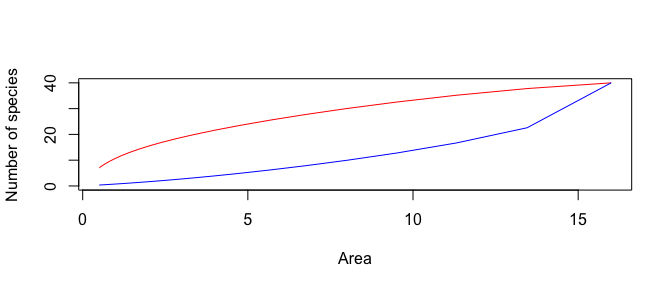Introduction

The package meteR is designed to facilitate fitting the models for the Maximum Entropy Theory of Ecology (METE) from data. For an overview of METE, see Harte et al. (2008), Harte (2011), and Harte and Newman (2014). Note that throughout this tutorial we use the notation from these sources without extensive explanation (Figure 1). meteR can use data input in multiple formats and predict all the fundamental distributions and relationships of the theory. Our objective is to facilitate tests of METE with empirical data sets.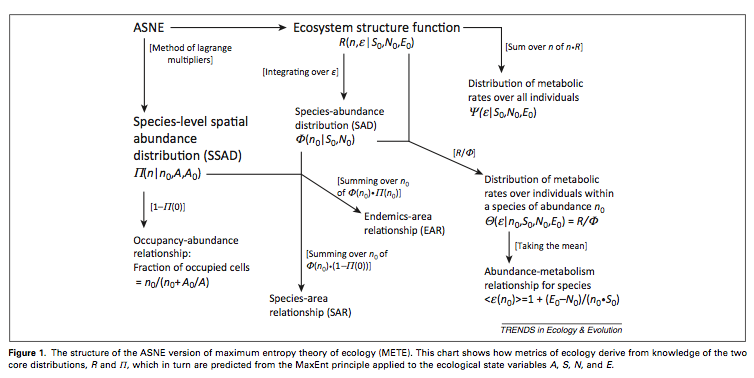fig1

Figure 1 was taken from Harte and Newman (2014) TREE 29: 384–389 and illustrates the derivation of, and connection between, different METE distributions and relationships.

Case Study 1 - Abundance and Power Distributions

We begin illustrating the capabilities of meteR with data from an arthropod community (Gruner 2007) collected via canopy fogging in Hawaii and including individual body mass measurements for each individual collected. These data are distributed as part of meteR. We will use it to illustrate the construction of distributions related to abundance and metabolic rate. Notably, these data do not contain any spatial information so we illustrate spatial predictions with a different data set in the next section.

library(meteR)
data(arth)
dim(arth)
##  547   3
##        spp count   mass
## 1 blacchel     1 4.7480
## 2 mecyocul     1 1.6490
## 3 eurynsp1     1 0.2584
## 4 eurynsp1     1 0.2584
## 5 eurynsp1     1 0.2584
## 6 eurynsp1     1 0.2584

This data set illustrates one data format used by meteR; each row represents an individual, with an observation of its metabolic rate (note that we convert mass to metabolic rate using the usual relationship of Metabolic Scaling Theory such that metabolic rate $$M \propto mass^{3/4}$$). If multiple individuals of the same size from the same species are observed (and lumped into one record), these can be specified as well, i.e., by letting arth$count be something other than 1. For an example of such formatting see the data set anbo, included with meteR, discussed in the next section. There are two main reasons to provide data to meteR: (1) meteR will calculate the state variables $$N_0$$ (number of individuals), $$S_0$$ (number of species), $$E_0$$ (total metabolic rate) and relevant summary statistics automatically; (2) these data are used by meter to compare against predictions. If data are not provided, the values for the state variables can be directly specified by the user (see Case Study 3). Analysis begins by building the ecosystem structure function (ESF; $$R(n,e)$$) from which all non-spatial macroecological metrics can be derived. $$R(n, e)$$ describes the joint probability of observing a species with $$n$$ individuals and a randomly chosen member of that species having metabolic rate $$e$$. METE computes this distribution by maximizing information entropy relative to the constraints of $$N_0/S_0$$ and $$E_0/S_0$$ using the method of Lagrange multipliers. In meteR we achieve this as follows: esf1 <- meteESF(spp=arth$spp,
abund=arth$count, power=arth$mass^(3/4),
minE=min(arth$mass^(3/4))) esf1 ## METE object with state variables: ## S0 N0 E0 ## 76.00 547.00 15868.26 ## ## with Lagrange multipliers: ## la1 la2 ## 0.037929267 0.004960427 str(esf1) ## List of 6 ##$ La       : Named num [1:2] 0.03793 0.00496
##   ..- attr(*, "names")= chr [1:2] "la1" "la2"
##  $La.info :List of 5 ## ..$ syst.vals: num [1:2] 8.88e-16 -2.84e-14
##   ..$converg : int 2 ## ..$ mesage   : chr "x-values within tolerance 'xtol'"
##   ..$nFn.calc : int 4 ## ..$ nJac.calc: int 1
##  $Z : Named num 639 ## ..- attr(*, "names")= chr "la2" ##$ state.var: Named num [1:3] 76 547 15868
##   ..- attr(*, "names")= chr [1:3] "S0" "N0" "E0"
##  $emin : num 0.0344 ##$ data     :'data.frame':   547 obs. of  3 variables:
##   ..$s: Factor w/ 76 levels "alapspu1","anagnigr",..: 8 38 27 27 27 27 27 27 54 21 ... ## ..$ n: int [1:547] 1 1 1 1 1 1 1 1 1 1 ...
##   ..$e: num [1:547] 93.4 42.3 10.5 10.5 10.5 ... ## - attr(*, "class")= chr "meteESF" Note that we use the terms ‘power’ and ‘metabolic rate’ interchangeably (units of power are energy/time and thus an energetic rate). Further, note that we specified the minimum value for the metabolic rate, but that the minimum observed value will be taken by default. Without loss of generality, metabolic rates are re-scaled by this minimum such that the minimum possible observable metabolic rate is 1. This is necessary for the underlying mathematics as discussed in Harte (2011). The returned object (of class meteESF) contains useful information. In addition to returning the inputs (in analogy, e.g., to common model fitting functions such as lm), it returns the Lagrange multipliers from entropy maximization as well as information about the fitting procedure. Species Abundance Distribution (SAD) From the ESF, we can predict macroecological patterns. We begin with the species abundance distribution (SAD). sad1 <- sad(esf1) sad1 ## Species abundance distribution predicted using raw data ## with parameters: ## S0 N0 E0 ## 76.00 547.00 15868.26 ## la1 la2 ## 0.03793 0.00496 The S3 method sad for class meteESF extracts the SAD and provides density (d), probability (p) and quantile (q) functions, as well as random number generation (r) for the distribution (see these with str(sad1)). This specification follows the conventions used by statistical distributions in the stats package (e.g. see ?rnorm). These distributions allow us to use the model in a number of ways. You can access these functions directly as follows; e.g. randomly generating samples from the fitted distribution or determining the quantiles. sad1$r(20)
##    6  2  3  1  2  1 64 20 27 12  3  3  2 10 12  3  3  1  4  6
sad1$q(seq(0,1,length=10)) ##  1 1 1 2 2 4 6 9 17 547 meteR readily plots the SAD as either a rank-abundance distribution (ptype=rad) or a cumulative distribution (ptype=cdf) (and other predicted distributions): par(mfrow=c(1,2)) plot(sad1, ptype='rad', log='y') plot(sad1, ptype='cdf', log='x')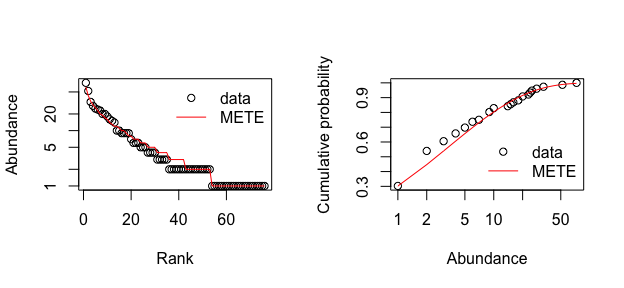Different ways of plotting the SAD meteR provides functions to assess model fit based on likelihood and residuals. First, we illustrate likelihood methods: #== calculate the liklihood of the data, given the fitted model logLik(sad1) ## 'log Lik.' -201.8189 (df=2) #== randomly generate 100 data sets from the fitted distribution and calculate #== the z-score of the data w.r.t. these simulations llz <- logLikZ(sad1, nrep=100, return.sim=TRUE) ## simulating data that conform to state variables: ## attempt 1 llz$z
## 'log Lik.' 0.1427271 (df=2)
#== plot the distributions
plot(density(llz$sim, from=0), xlim=range(c(llz$sim,llz$z)), xlab='Scaled log(likelihood)',col='red') #== add 95% quantile region abline(v=quantile(llz$sim, 0.95), col='red')
#== add observed likelihood
abline(v=llz$z,lty=2) legend('top',legend=c('data','simulated from METE'), col=c('black','red'), lty=c(2, 1), bg='white')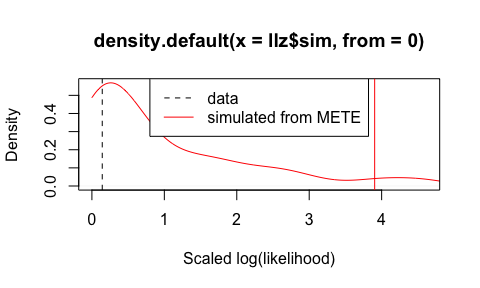Likelihood-based z-score. Because the likelihood of the observed data falls within the 95% quantile region of the simulated likelihoods, we have confidence that the model provides a good fit. It should be noted that the z-score and associated simulation values are square-transformed such that the resulting null distribution approaches a Chi-squared distribution with d.f. = 1. Specifically the z-score is $$z = ((\text{logLik}_{obs} - mean(\text{logLik}_{sim})) / sd(\text{logLik}_{sim}))^2$$. Note that other utilities that extract liklihoods from model objects are also useful, including AIC, thus opening up all model comparison capabilities in R and its contributed packages: AIC(sad1) ##  407.6378 Next, we illustrate methods relying on residuals to assess model fit. Residuals can be calculated either on the rank abundance (type='rank') or cumulative (type='cumulative') distribution and can be calculated as relative residuals ($$(x_i - \hat{x})/\hat{x}$$) or absolute ($$x_i - \hat{x}$$) by setting argument relative to TRUE or FALSE, respectively. Relative residuals can be thought of as the proportional difference between observed and expected abundance or probability. From the residuals, mean squared error can be calculated and used to evaluate model fit. Although both rank abundance and cumulative density options are available, we recommend only using rank abundance as cumulative density is constrained at upper and lower bounds and so its residuals will not be as reliable. #== calculate the residuals from the fitted distribution head(residuals(sad1)) ## blacfrau eurynsp1 mecymolo sierspuM anysgsu1 oligspu1 ## 0.19672131 0.20930233 -0.02941176 -0.03448276 -0.03846154 0.04347826 head(residuals(sad1, type='cumulative', relative=FALSE)) ##  0.003392984 -0.002220492 0.008352198 0.008744914 0.006623537 ##  -0.002216539 #== calculate the mean-squared error mse(sad1, type='rank', relative=FALSE) ##  3.881579 #== randomly generate 100 data sets from the fitted distribution and calculate #== the z-score of the data w.r.t. these simulations msez.rank <- mseZ(sad1, nrep=100, return.sim=TRUE, type='rank') ## simulating data that conform to state variables: ## attempt 1 msez.rank$z
##  0.6157085
#== plot the distributions
plot(density(msez.rank$sim), xlim=c(0.05, 10), xlab='Scaled mean squared error',col='red', log='x') ## Warning in xy.coords(x, y, xlabel, ylabel, log): 11 x values <= 0 omitted ## from logarithmic plot #== add 95% quantile region abline(v=quantile(msez.rank$sim, 0.95), col='red')
#== add observed likelihood
abline(v=msez.rank$z,lty=2) legend('top',legend=c('data','simulated from METE'), col=c('black','red'), lty=c(2 ,1),bg='white')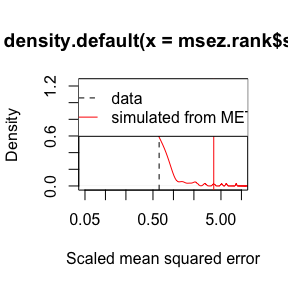Here again we fail to reject METE as the model that generated the observed data (the observed MSE is below the 95% quantile region). We can also see that the distribution of errors (which again uses the same square transformation as in logLikZ) is not as Chi-squared shaped as the distribution of likelihoods. Thus although residuals can be useful (specifically we can see whether, e.g., common species are more or less common than predicted by METE) we recommend using likelihood for evaluating model fit. Individual Power Distribution (IPD) Similarly to the analyses illustrated above for the SAD, one can examine the individual power distribution (IPD; the distribution of metabolic rates, or power, over individuals in the community). We illustrate with an abbreviated version of the analyses above. First, fit and plot the IPD. ipd1 <- ipd(esf1) ipd1 ## Individual metabolic rate distribution predicted using raw data ## with parameters: ## S0 N0 E0 ## 76.00 547.00 15868.26 ## la1 la2 ## 0.03793 0.00496 str(ipd1) # analogous structure to sad1 above ## List of 8 ##$ type     : chr "ipd"
##  $data : num [1:547] 178 178 153 129 119 ... ##$ d        :function (epsilon, log = FALSE)
##  $p :function (epsilon, lower.tail = TRUE, log.p = FALSE) ##$ q        :function (p, lower.tail = TRUE, log.p = FALSE)
##  $r :function (n) ##$ state.var: Named num [1:3] 76 547 15868
##   ..- attr(*, "names")= chr [1:3] "S0" "N0" "E0"
##  $La : Named num [1:2] 0.03793 0.00496 ## ..- attr(*, "names")= chr [1:2] "la1" "la2" ## - attr(*, "class")= chr [1:2] "ipd" "meteDist" ipd1$r(8) # random number generation from fitted distribution
##   17.619164  45.404053  24.245709 410.893816   2.416382   1.802014
##   17.068223  17.333033
par(mfrow=c(1,2))
plot(ipd1, ptype='cdf', log='x')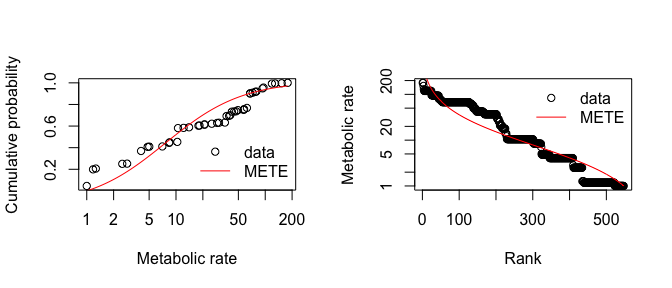Different ways of plotting the IPD

Next, assess the fit of the IPD.

##  -0.7704076 -0.6834267 -0.6735534 -0.6831556 -0.6739310 -0.6409113
logLik(ipd1)
## 'log Lik.' -2289.101 (df=2)
logLikZ(ipd1, nrep=100)
## simulating data that conform to state variables:
## attempt 1
## $z ## 'log Lik.' 7.011331 (df=2) ## ##$sim
## NULL
llz <- logLikZ(ipd1, nrep=100, return.sim=TRUE)
## simulating data that conform to state variables:
## attempt 1
plot(density(llz$sim),xlim=range(c(llz$sim,llz$obs)),xlab='log(likelihood)',col='red') abline(v=llz$obs,lty=2)
llz$z ## 'log Lik.' 5.242685 (df=2) legend('top',legend=c('data','simulated\nfrom METE'),col=c('black','red'), lty=c(1,1),bty='n')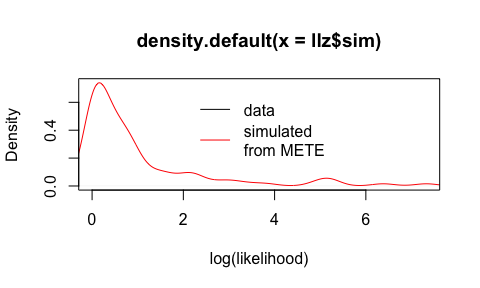Likelihood-based z-score. Interestingly, although it appears that the data are unlikely to have been drawn from the METE distribution based on the z-score of the likelihood, the mean squared error suggests otherwise. mse(ipd1, type='rank', relative=FALSE) ##  1915.809 mseZ <- mseZ(ipd1, nrep=100, return.sim=TRUE) ## simulating data that conform to state variables: ## attempt 1 mseZ$z
##  97.49209
plot(density(mseZ$sim),xlim=range(c(mseZ$sim,mseZ$obs)),xlab='mse',col='red') abline(v=mseZ$obs,lty=2)
legend('top',legend=c('data','simulated\nfrom METE'),col=c('black','red'),
lty=c(1,1),bty='n')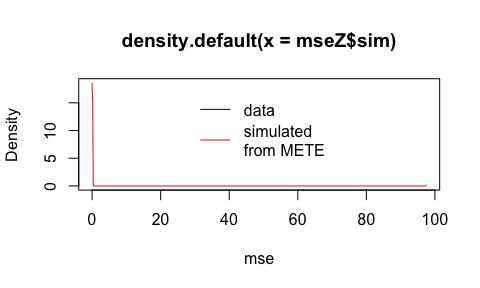Mean squared error-based z-score.

METE predicts two other distributions relating to metabolic rates: the distribution of metabolic rates across individuals within a species with $$n$$ total individuals (the sipd, $$\Theta(e | n)$$ in the notation of Harte 2011) and the species level distribution of average metabolic rates (the spd, $$\nu(e)$$ in the notation of Harte 2011).

We can obtain the SIPD with S3 method sipd which requires either a species ID be specified or the total abundance of a hypothetical species:

sipd1 <- sipd(esf1, sppID=27)
plot(sipd1, log='x',ylim=c(0,1))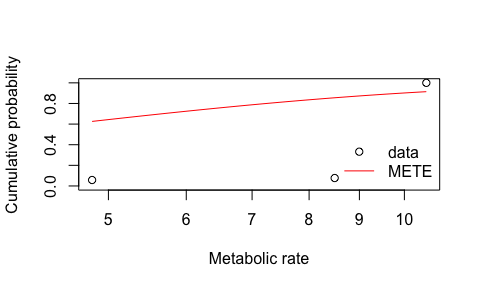Distribution of metabolic rates of individuals in a species with $$n$$ individuals

## sipd based on total abundance of a hypothetical species
sipd2 <- sipd(esf1, n=25)

Note that this plot is not particularly informative in this case because there are only 3 unique observations of metabolic rate for this species.

We can obtain the SPD with S3 method spd:

spd1 <- spd(esf1)
plot(spd1, log='x')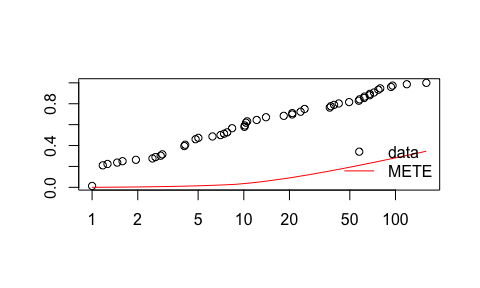Distribution of metabolic rates of individuals in a species with $$n$$ individuals

Because the distribution of within species metabolic rates depends on the total abundance of that species, it makes sense that there should be a relationship between the mean metabolic rate and the abundance of a species. This relationship has been widely studied (Damuth 1998; White et al. 2007) and if mean metabolic rate is independent of $$n$$ this is known as energy equivalence. To explore this relationship we introduce a new S3 class meteRelat which acts like meteDist to house both observed and theoretical predictions. meteRelat objects represent a deterministic relationship, in contrast to the probabilistic distributions represented by meteDist objects. To obtain the relationship between abundance and mean metabolic rate we use the ebar function:

ebar1 <- ebar(esf1)
plot(ebar1)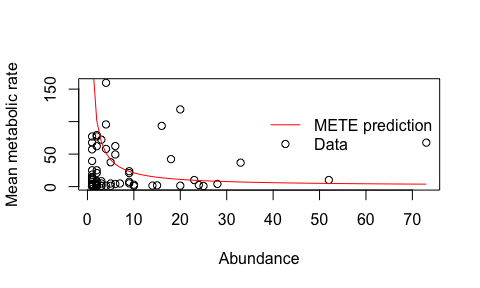Relationship between abundance and mean metabolic rate

Case Study 2 - Spatial distributions

Next, we illustrate spatial analyses with data from a survey of a desert annual grassland community at the Anza Borrego Preserve (Harte and Harte, unpub. data). The survey recorded every herbaceous plant within a 4x4m plot gridded to ever square meter (resulting in 16 total cells). Thus spatial data take the form of the row and column identity where each plant was recorded. This dataset is distributed as part of meteR. We will use it to illustrate the construction of distributions related to abundance and area. Note that metabolic rate/body mass information are not available, but that we can still analyze many of METE’s predictions related to abundance and spatial distribution. As shown in case study 1 above, we can build the ESF and investigate the SAD.

data(anbo)
##   row column    spp count
## 1   3      3   cabr     3
## 2   3      3 caspi1    20
## 3   3      3   crcr     3
## 4   3      3  crsp2     1
## 5   3      3   gnwe    11
## 6   3      3  grass    11
esf2 <- meteESF(spp=anbo$spp, abund=anbo$count)
str(esf2)
## List of 6
##  $La : Named num [1:2] 0.00037 0.00109 ## ..- attr(*, "names")= chr [1:2] "la1" "la2" ##$ La.info  :List of 5
##   ..$syst.vals: num [1:2] 1.04e-10 1.04e-10 ## ..$ converg  : int 2
##   ..$mesage : chr "x-values within tolerance 'xtol'" ## ..$ nFn.calc : int 3
##   ..$nJac.calc: int 1 ##$ Z        : Named num 5973
##   ..- attr(*, "names")= chr "la2"
##  $state.var: Named num [1:3] 24 2445 NA ## ..- attr(*, "names")= chr [1:3] "S0" "N0" "E0" ##$ emin     : num 1
##  $data :'data.frame': 2445 obs. of 2 variables: ## ..$ s: Factor w/ 24 levels "arsp1","cabr",..: 2 2 2 3 3 3 3 3 3 3 ...
##   ..$n: num [1:2445] 1 1 1 1 1 1 1 1 1 1 ... ## - attr(*, "class")= chr "meteESF" Note that when metabolic rate data are absent, meteR assumes a very large value for the state variable E0, such that terms involving E0 can be safely ignored in distributions and relationships involving abundance following Harte (2011). (sad2=sad(esf2)) ## Species abundance distribution predicted using raw data ## with parameters: ## S0 N0 E0 ## 24 2445 NA ## la1 la2 ## 0.00037 0.00109 par(mfrow=c(1,2)) plot(sad2, ptype='rad') plot(sad2, ptype='cdf')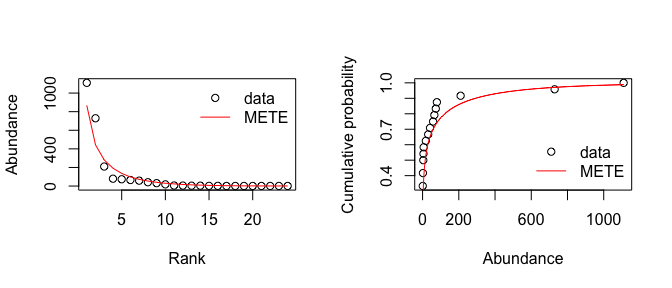Different ways of plotting the SAD for the anbo data. Analogous to the construction of the ESF above, we construct the Spatial Structure Function (SSF) (denoted $$\Pi(n | n_0, A, A_0)$$ in Harte 2011). $$\Pi$$ depends on the total abundance $$n_0$$ of the species of interest as well as the the total area of the study system $$A_0$$ and the scale for which the SSF is to be calculated ($$A$$). Again, in analogy to how the SAD is constructed from the ESF, we can obtain the Species Spatial Abundance Distribution (SSAD) from the SSF, but here must specify the species for which the SSF and SSAD are to be constructed as well as the spatial state variables (both the area of interest A and the total area A0). ## note we are calculating SSF for the species crcr pi1 <- meteSSF(anbo$spp, 'crcr', anbo$count, row=anbo$row, col=anbo$column, A=1, A0=16) pi1 ## METE object with state variables: ## n0 A A0 ## 65 1 16 ## ## with Lagrange multipliers: ##  0.2200619 plot(ssad(pi1))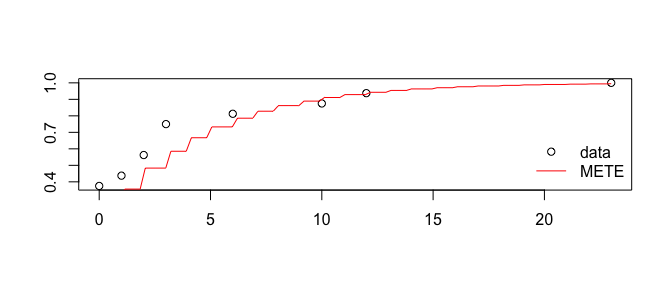SSAD for the anbo data. Areas greater than the minimum can also be explored: pi2 <- meteSSF(anbo$spp, 'crcr', anbo$count, row=anbo$row, col=anbo$column, A=2, A0=16) pi2 ## METE object with state variables: ## n0 A A0 ## 65 2 16 ## ## with Lagrange multipliers: ##  0.1156423 plot(ssad(pi2)) # theory is not looking too good for this case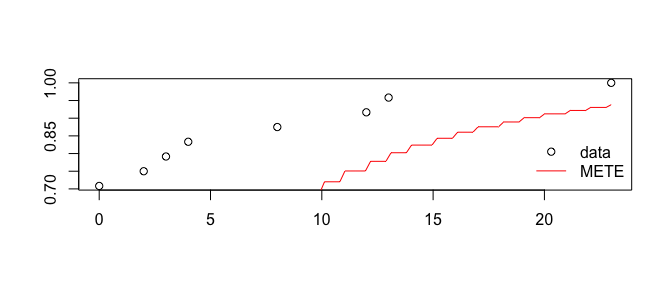SSAD for A=2. This is done by aggregating cells internally in meteSSF. We will examine the case where spatial data are given in the form of x and y coordinates for each abundance measure by simulating such data. ## jitter abundance records within each cell anbo$x <- runif(nrow(anbo), 0, 1) + anbo$column anbo$y <- runif(nrow(anbo), 0, 1) + anbo$row pi3 <- meteSSF(anbo$spp, 'crcr', anbo$count, x=anbo$x, y=anbo$y, A=1, A0=16) plot(ssad(pi3)) # the plot has naturally changed slightly due to the jittering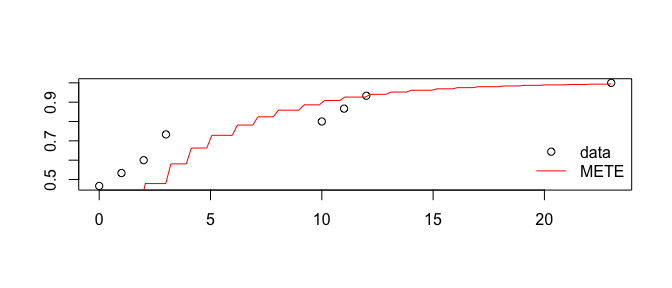SSAD for simulated x,y anbo data. Next we predict the Species Area Relationship and Endemics Area Relationship (SAR and EAR). We begin by predicting the number of species at scales smaller than the total plot size. For this we need to combine the ESF and the SSF. Note the the EAR is obtained in all functions by specifying argument EAR=TRUE. Downscaling assumes that METE holds at the largest spatial scale and smaller scales are spatially nested subsamples of this larger scale. As such this approach makes intuitive sense for small-scale plot data. Conversely upscaling allows one to use plot-level data to predict species richness at scales much larger than the surveyed plot. This is achieved by iteratively solving for the larger spatial scale that would yield the observed smaller scale under nested subsampling. This iterative process can be carried out to indefinite scales. The METE-predicted SAR can be obtained in two ways, one directly and one recalling the meteDist objects explored earlier. ## first the direct method anbo.esf <- meteESF(spp=anbo$spp, abund=anbo$count) anbo.thr.downscale <- downscaleSAR(anbo.esf, 2^(seq(-3, 4, length=17)), 16) anbo.thr.downscale ## theoretical species area relationship ranging from ## A: [0.125, 16] ## S: [5.63177023121553, 23.9999999999845] anbo.thr.downscaleEAR <- downscaleSAR(anbo.esf, 2^(seq(-3, 4, length=17)), 16, EAR=TRUE) ## upscaling anbo.thr.upscale <- upscaleSAR(anbo.esf, 16, 2^6) ## plotting plot(anbo.thr.downscale, xlim=c(1, 2^6), ylim=c(0, 35)) plot(anbo.thr.downscaleEAR, col='blue', add=TRUE) plot(anbo.thr.upscale, col='red', add=TRUE)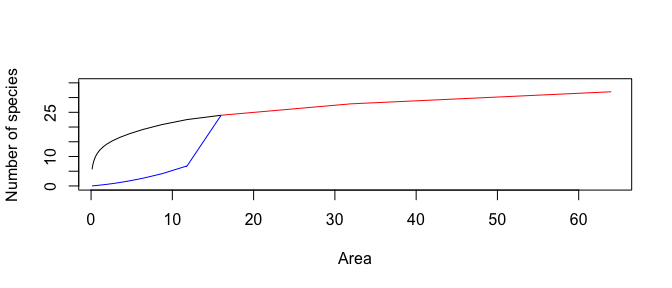The SAR (black) and EAR (blue) for the anbo data. In this example, there are 24 species distributed across 16 quadrats, hence the downscaled SAR (black) and EAR (blue) converge at 24 species at an area of 16. Endemics here must be interpreted as local endemics, thus all 24 species are endemic’’ to the 16m$$^2$$ plot. Upscale species richness is shown in red. Note that for mathematical convenience (discussed in Harte 2011) we only consider doublings of area when computing upscaled species richness. For intermediate areas one can simply interpolate. To compare the mete prediction with data we use meteSAR to obtain a meteRelat object that stores both observed data and theoretical predictions: anbo.sar <- meteSAR(anbo$spp, anbo$count, anbo$row, anbo$col, Amin=1, A0=16) anbo.sar ## Species area relationship predicted using raw data ## theoretical species area relationship ranging from ## A: [1, 16] ## S: [11.9430546490289, 23.9999999999746] ## empirical species area relationship ranging from ## A: [1, 16] ## S: [3, 24] plot(anbo.sar, log='xy')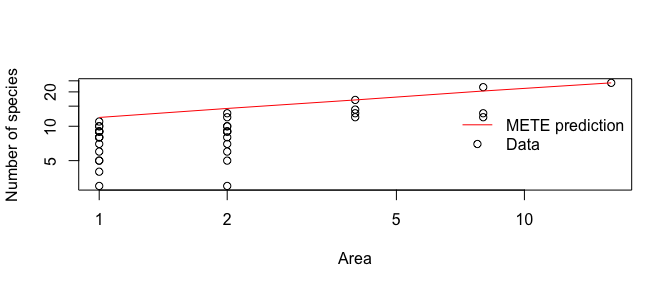Comparing SAR with data. Note that we can also calculate the SAR or EAR using x, y data instead of rows and columns similarly to how we did this with the SSF: anbo.sar.sim <- meteSAR(anbo$spp, anbo$count, x=anbo$x, y=anbo$x, Amin=1, A0=16) anbo.sar.sim ## Species area relationship predicted using raw data ## theoretical species area relationship ranging from ## A: [1.052128690952, 8.41702952761597] ## S: [12.1586431148625, 20.7090489525889] ## empirical species area relationship ranging from ## A: [1.052128690952, 8.41702952761597] ## S: [7, 22] The empirical SAR (or EAR) can also be directly obtained from the data without jointly calculating the theoretical predictions: ## empirical SAR and EAR anbo.obs.sar <- empiricalSAR(anbo$spp, anbo$count, anbo$row, anbo$col, Amin=1, A0=16) plot(anbo.obs.sar)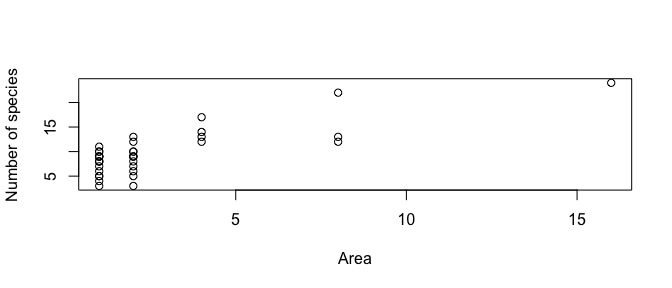anbo.obs.ear <- empiricalSAR(anbo$spp, anbo$count, anbo$row, anbo$col, Amin=1, A0=16, EAR=TRUE) plot(anbo.obs.ear)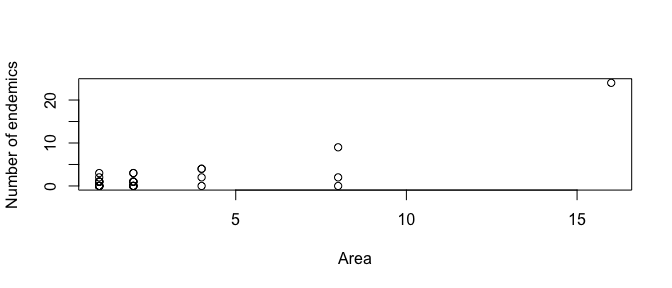Case Study 3 - Specifying the state variables meteR allows the user to manually specify the state variables, which can be useful to explore how METE predictions vary as a function of state variables without regard to specific datasets. esf3 <- meteESF(N0=4000, S0=50, E0=1e5, minE=1) sad3 <- sad(esf3) ipd3 <- ipd(esf3) par(mfrow=c(1,2)) plot(sad3) ## Warning in max(plot.par$xlim): no non-missing arguments to max; returning -
## Inf
plot(ipd3)
## Warning in max(plot.par\$xlim): no non-missing arguments to max; returning -
## Inf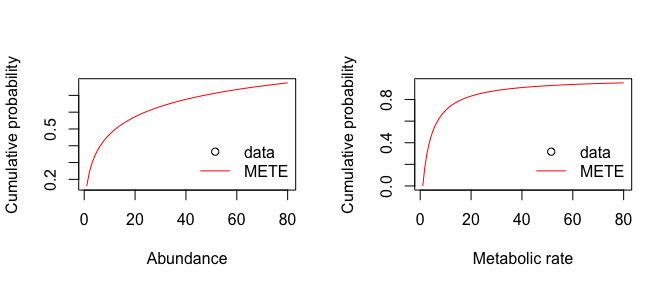Similarly, one can predict spatial distributions from the state variables. First, we downscale the species area relationship.

## theoretical SARs from state variables only
thr.downscale <- downscaleSAR(meteESF(S0=40, N0=400), 2^seq(-1, 4, by=0.25), 16)
thr.downscaleEAR <- downscaleSAR(meteESF(S0=40, N0=400), 2^seq(-1, 4, by=0.25), 16, EAR=TRUE)
plot(thr.downscale, ylim=c(0, 40), col='red')